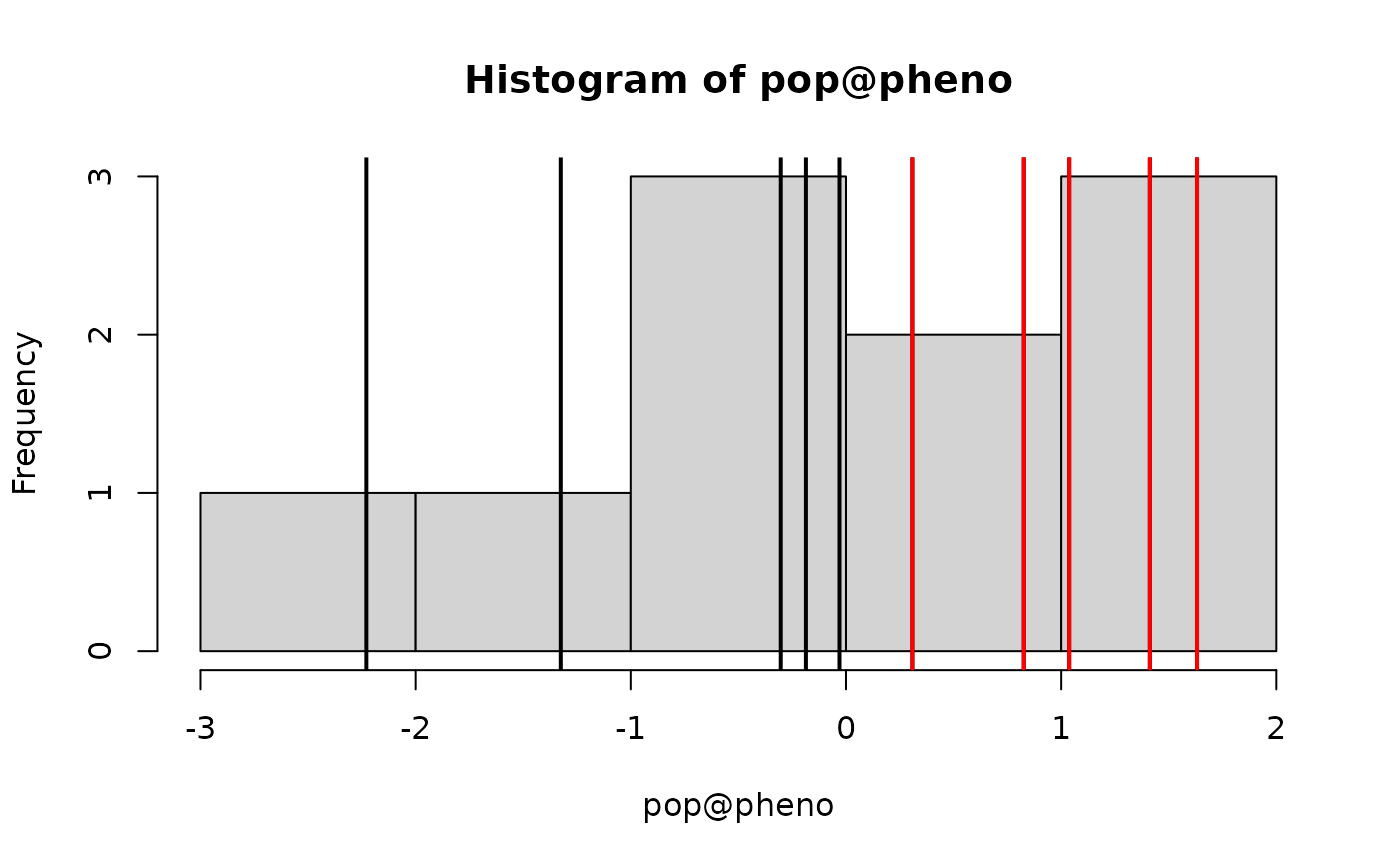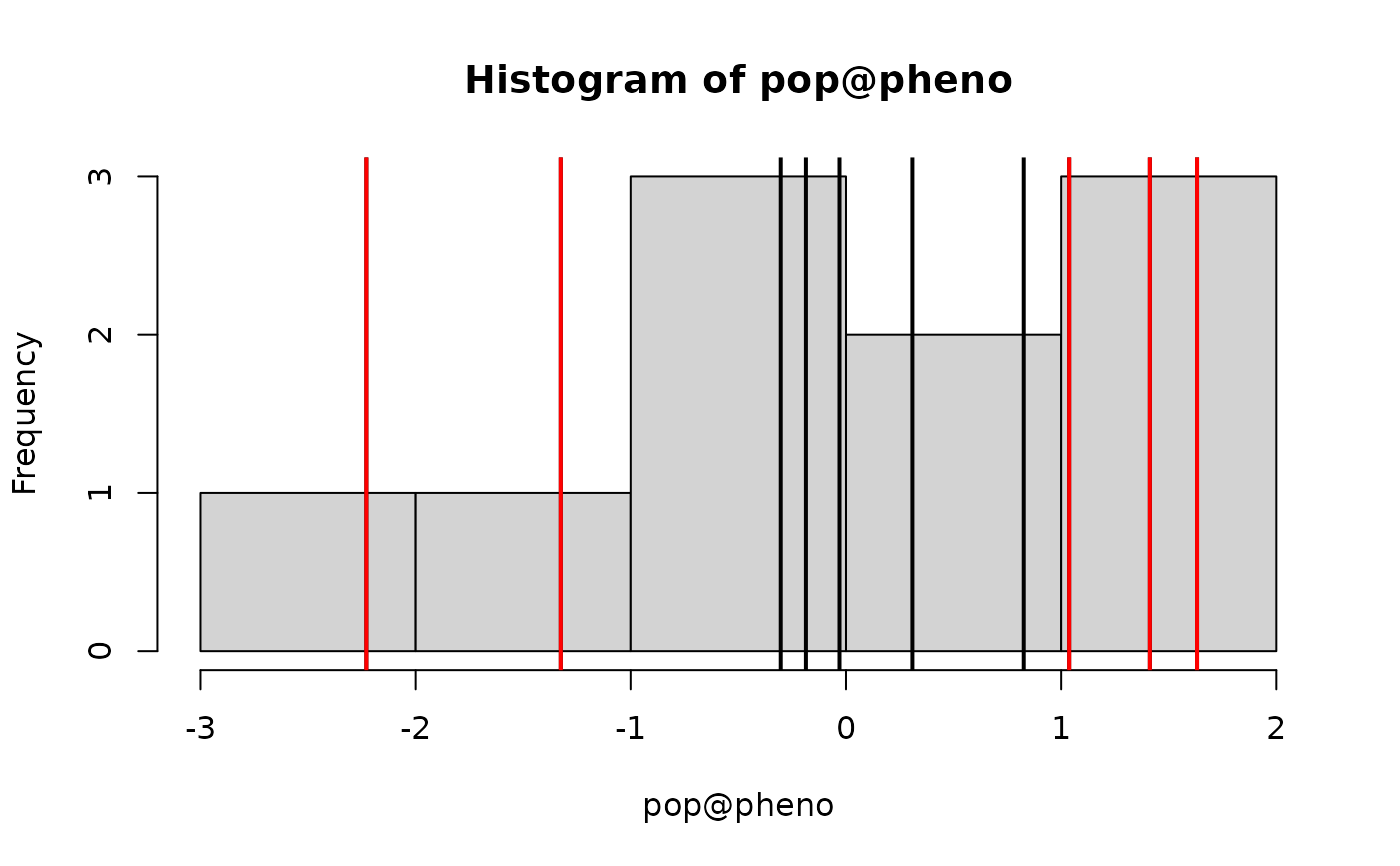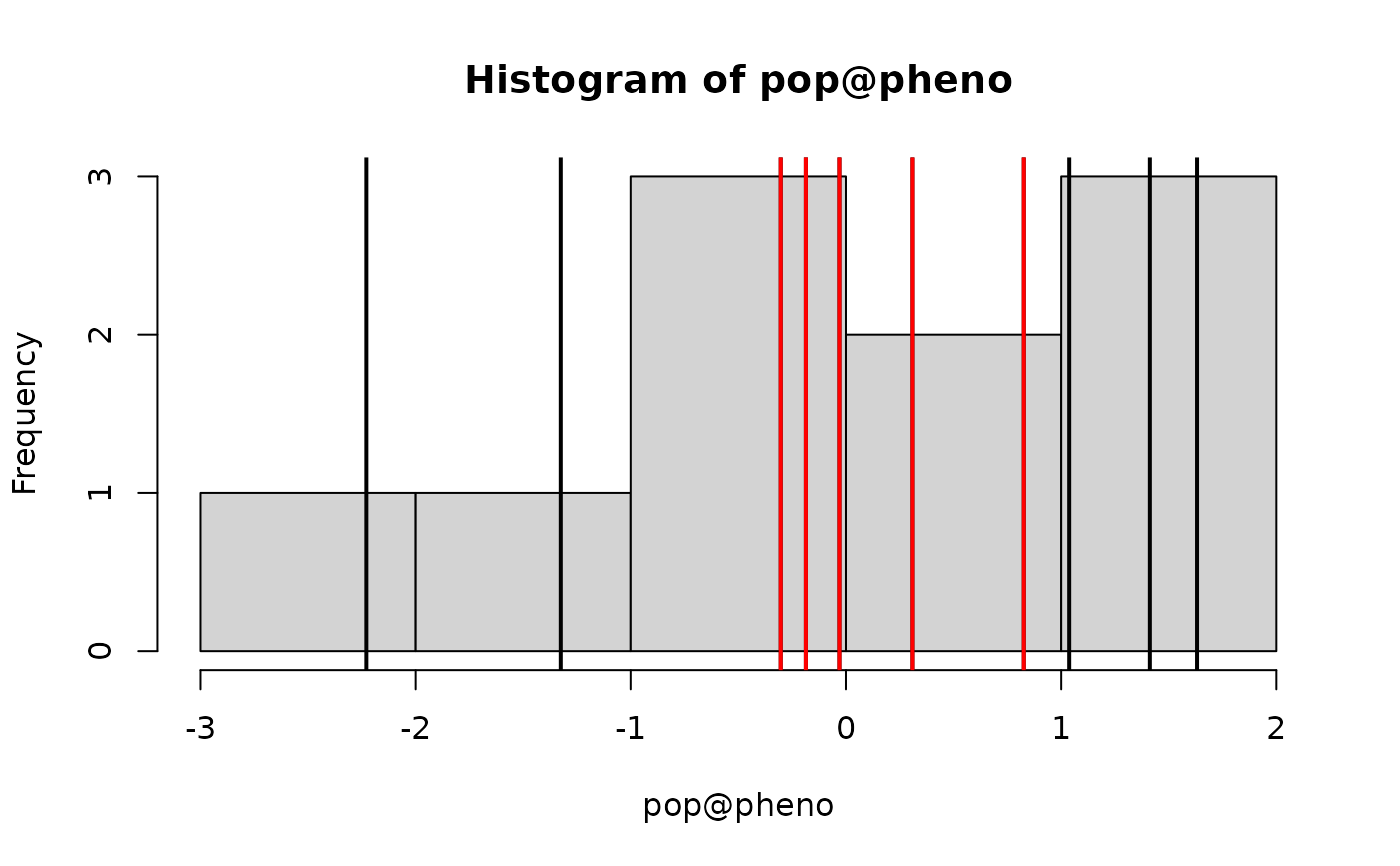Selects a subset of nInd individuals from a population.

## Usage

selectInd(
pop,
nInd,
trait = 1,
use = "pheno",
sex = "B",
selectTop = TRUE,
returnPop = TRUE,
candidates = NULL,
simParam = NULL,
...
)

## Arguments

pop

and object of Pop-class, HybridPop-class or MultiPop-class

nInd

the number of individuals to select

trait

the trait for selection. Either a number indicating a single trait or a function returning a vector of length nInd. The function must work on a vector or matrix of use values. See the examples and selIndex.

use

select on genetic values "gv", estimated breeding values "ebv", breeding values "bv", phenotypes "pheno", or randomly "rand"

sex

which sex to select. Use "B" for both, "F" for females and "M" for males. If the simulation is not using sexes, the argument is ignored.

selectTop

selects highest values if true. Selects lowest values if false.

returnPop

should results be returned as a Pop-class. If FALSE, only the index of selected individuals is returned.

candidates

an optional vector of eligible selection candidates.

simParam

an object of SimParam

...

additional arguments if using a function for trait

## Value

Returns an object of Pop-class, HybridPop-class or MultiPop-class

## Examples

#Create founder haplotypes
founderPop = quickHaplo(nInd=10, nChr=1, segSites=10)

#Set simulation parameters
SP = SimParam$new(founderPop) SP$nThreads = 1L
SP$addTraitA(10) SP$setVarE(h2=0.5)

#Create population
pop = newPop(founderPop, simParam=SP)

#Select top 5 (directional selection)
pop2 = selectInd(pop, 5, simParam=SP)
hist(pop@pheno); abline(v = pop@pheno, lwd = 2)
abline(v = pop2@pheno, col = "red", lwd = 2)#Select 5 most deviating from an optima (disruptive selection)
squaredDeviation = function(x, optima = 0) (x - optima)^2
pop3 = selectInd(pop, 5, simParam=SP, trait = squaredDeviation, selectTop = TRUE)
hist(pop@pheno); abline(v = pop@pheno, lwd = 2)
abline(v = pop3@pheno, col = "red", lwd = 2)#Select 5 least deviating from an optima (stabilising selection)
pop4 = selectInd(pop, 5, simParam=SP, trait = squaredDeviation, selectTop = FALSE)
hist(pop@pheno); abline(v = pop@pheno, lwd = 2)
abline(v = pop4@pheno, col = "red", lwd = 2)